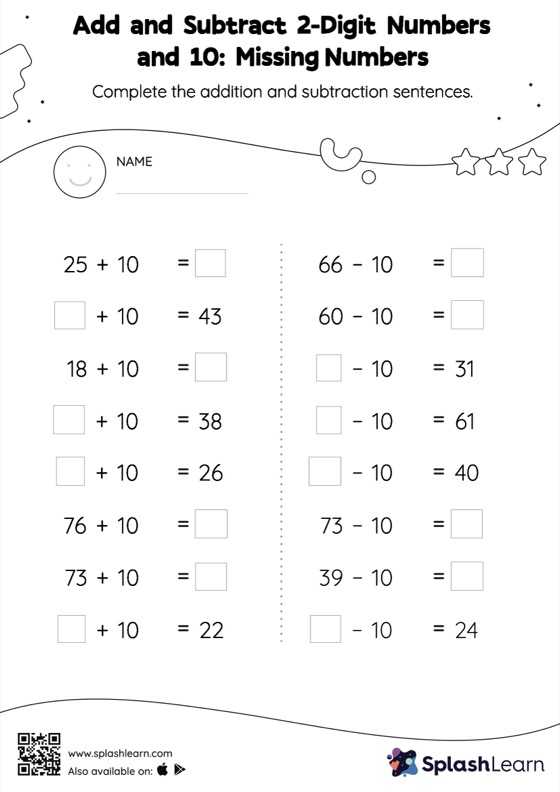# Add and Subtract 2-Digit Numbers and 10: Missing Numbers Worksheet

Home > Add and Subtract 2-Digit Numbers and 10: Missing NumbersThis worksheet challenges young mathematicians to hone their math skills by solving a set of problems on adding and subtracting 2-digit numbers and 10. This worksheet is about practicing with the horizontal format in which numbers are written side by side. To develop flexibility with numbers and operations, students need to have enough practice in this format and not just rely on the vertical/column method.